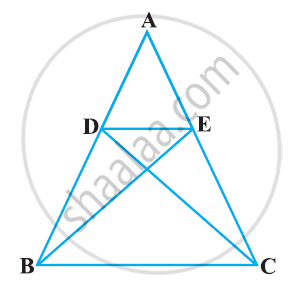# In the following figure, if ΔABE ≅ ΔACD, show that ΔADE ∼ ΔABC. - Mathematics

In the following figure, if ΔABE ≅ ΔACD, show that ΔADE ∼ ΔABC.#### Solution

It is given that ΔABE ≅ ΔACD.

∴ AB = AC [By CPCT] (1)

And, AD = AE [By CPCT] (2)

(AD)/(AB) = (AE)/(AC)   [Dividing equation 2 by 1]

A = ∠A [Common angle]

∴ ΔADE ∼ ΔABC [By SAS similarity criterion]

Concept: Criteria for Similarity of Triangles
Is there an error in this question or solution?

#### APPEARS IN

NCERT Class 10 Maths
Chapter 6 Triangles
Exercise 6.3 | Q 6 | Page 140